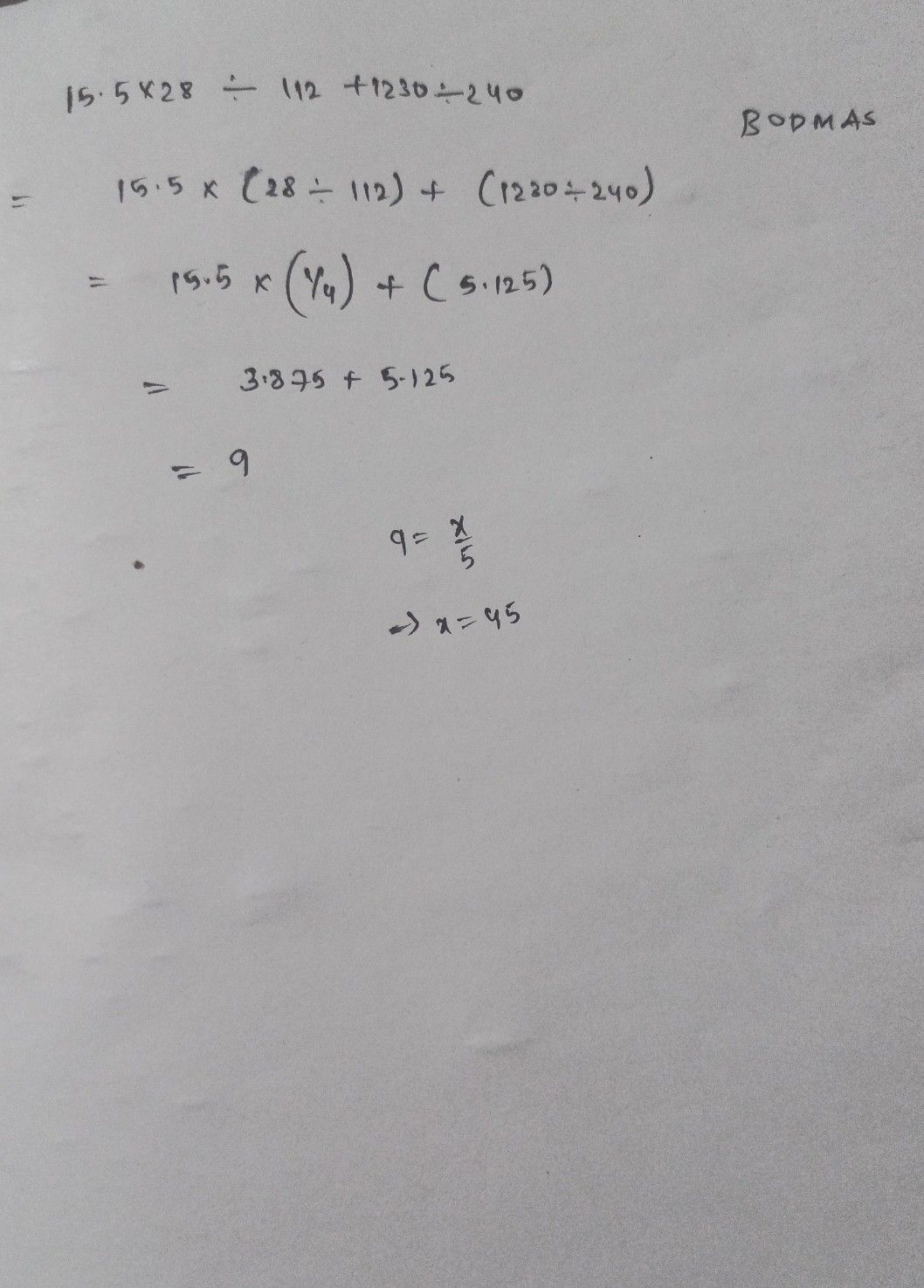Symbol
Problem$+=$ $Q:12$ $1s.5\times 28$ $\div$ $+$ $-2y0=2\div s$